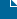In Uncategorized on March 30, 2010 by Matt Giuca

I wrote it this morning. I wasn’t sure if it could be done.

Since Haskell is a pure language, you can’t mutate things. That makes it tricky to construct cyclic data structures, but you can using Haskell’s laziness. A quick example is the cycle function in the Haskell prelude, defined thusly:

```cycle            :: [a] -> [a]
cycle []          = error "Prelude.cycle: empty list"
cycle xs          = xs' where xs'=xs++xs'
```

Note that you could write “cycle xs = xs ++ cycle xs”, but that isn’t strictly a cyclic list, it’s an infinite list. There is no semantic difference, only a very subtle operational one.

I used the same trick to create a full doubly-linked list (note that you can’t update this data structure, you can just create one from a singly-linked list and explore it as you wish).

```data DoubleList a = Cons (DoubleList a) a (DoubleList a)
| Nil

instance Show a => Show (DoubleList a) where
show Nil = "Nil"
show (Cons left x right) = "Cons <left> " ++ show x ++ " " ++ show right

fromList :: [a] -> DoubleList a
fromList xs = fromList' Nil xs
where
fromList' :: DoubleList a -> [a] -> DoubleList a
fromList' _ [] = Nil
fromList' prev (x:xs) = self
where self = Cons prev x (fromList' self xs)

interactive :: Show a => DoubleList a -> IO ()
interactive Nil = putStrLn "Empty list"
interactive (Cons left x right) = do
putStr \$ show x ++ " (l, r)> "
prompt <- getLine
case prompt of
"l" -> goto left
"r" -> goto right
_ -> interactive (Cons left x right)
where
goto Nil = do
putStrLn "End of list"
interactive (Cons left x right)
goto target = interactive target```

The show function doesn’t show the left side, because that would create an infinite string. But you can use the “interactive” function to explore the list interactively.